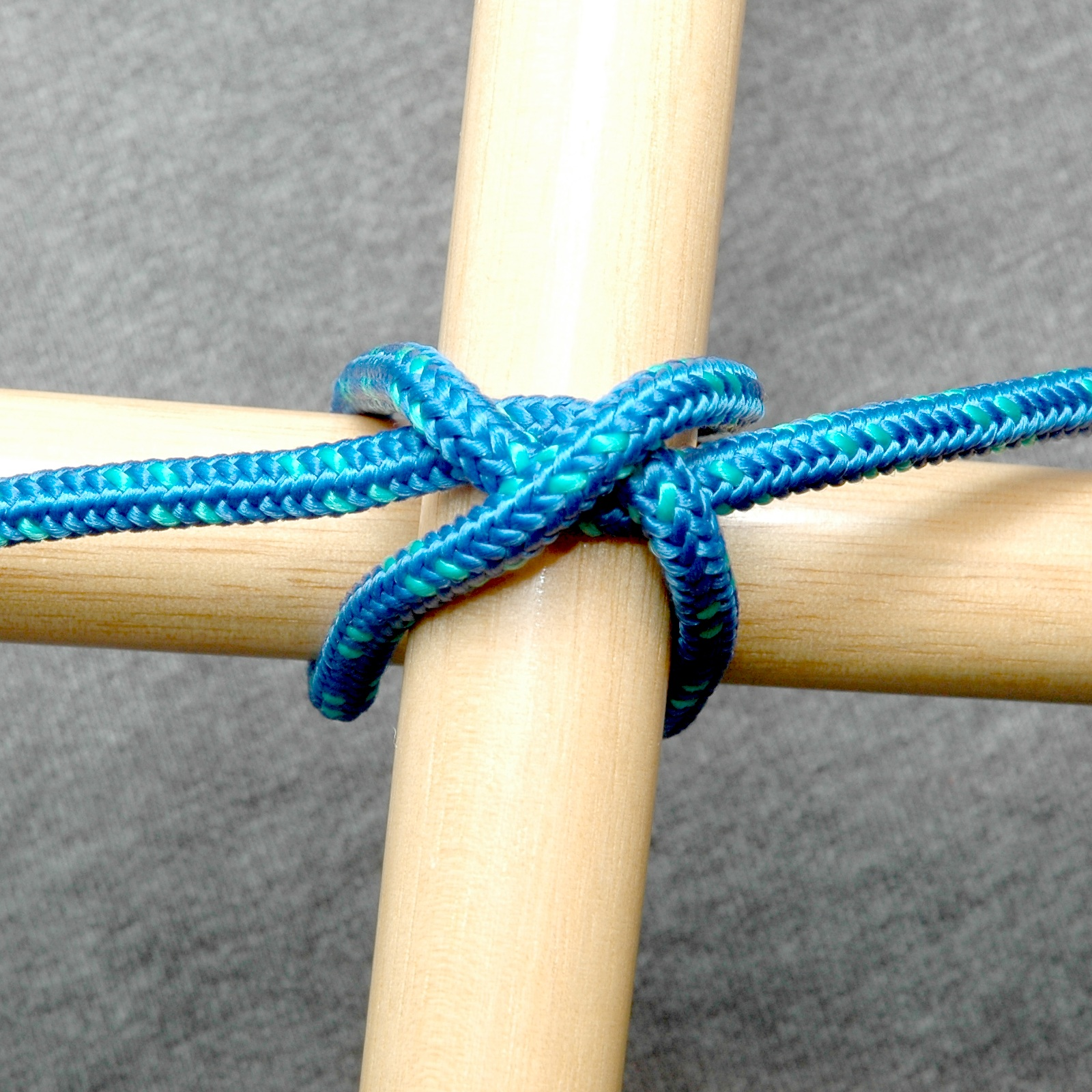# Transom knot

Transom knot

Knot-details
name=Transom knotcaption=
names=
type=lashing
strength=
origin=
related=Constrictor knot
releasing= Jamming
uses=Light-duty right-angle lashing
caveat=
abok_number=#385, #1182, #1255

The Transom knot is a simple lashing knot, based on the Constrictor knot, used to secure two linear objects at right angles to each other. To reinforce, a second Transom knot can be made on the opposite side and at a right-angle to the first. Suggested for binding kite sticks.Clifford W. Ashley, "The Ashley Book of Knots" (New York: Doubleday, 1944), 225.]

References

Wikimedia Foundation. 2010.

### Look at other dictionaries:

• Constrictor knot — Left: Constrictor knot Right: Double constrictor knot Names Constrictor knot, gunner s knot Category Binding …   Wikipedia

• Square knot — Square Square (skw[^a]r), a. 1. (Geom.) Having four equal sides and four right angles; as, a square figure. [1913 Webster] 2. Forming a right angle; as, a square corner. [1913 Webster] 3. Having a shape broad for the height, with rectilineal and… …   The Collaborative International Dictionary of English

• main transom — Wing Wing, n. [OE. winge, wenge; probably of Scand. origin; cf. Dan. & Sw. vinge, Icel. v[ae]ngr.] [1913 Webster] 1. One of the two anterior limbs of a bird, pterodactyl, or bat. They correspond to the arms of man, and are usually modified for… …   The Collaborative International Dictionary of English

• Wing transom — Wing Wing, n. [OE. winge, wenge; probably of Scand. origin; cf. Dan. & Sw. vinge, Icel. v[ae]ngr.] [1913 Webster] 1. One of the two anterior limbs of a bird, pterodactyl, or bat. They correspond to the arms of man, and are usually modified for… …   The Collaborative International Dictionary of English

• Glossary of nautical terms — This is a glossary of nautical terms; some remain current, many date from the 17th 19th century. See also Wiktionary s nautical terms, Category:Nautical terms, and Nautical metaphors in English. Contents: A B C D E F G H I J K L M N O P Q R …   Wikipedia

• Five-square — Square Square (skw[^a]r), a. 1. (Geom.) Having four equal sides and four right angles; as, a square figure. [1913 Webster] 2. Forming a right angle; as, a square corner. [1913 Webster] 3. Having a shape broad for the height, with rectilineal and… …   The Collaborative International Dictionary of English

• Square — (skw[^a]r), a. 1. (Geom.) Having four equal sides and four right angles; as, a square figure. [1913 Webster] 2. Forming a right angle; as, a square corner. [1913 Webster] 3. Having a shape broad for the height, with rectilineal and angular rather …   The Collaborative International Dictionary of English

• Square foot — Square Square (skw[^a]r), a. 1. (Geom.) Having four equal sides and four right angles; as, a square figure. [1913 Webster] 2. Forming a right angle; as, a square corner. [1913 Webster] 3. Having a shape broad for the height, with rectilineal and… …   The Collaborative International Dictionary of English

• Square measure — Square Square (skw[^a]r), a. 1. (Geom.) Having four equal sides and four right angles; as, a square figure. [1913 Webster] 2. Forming a right angle; as, a square corner. [1913 Webster] 3. Having a shape broad for the height, with rectilineal and… …   The Collaborative International Dictionary of English

• Square number — Square Square (skw[^a]r), a. 1. (Geom.) Having four equal sides and four right angles; as, a square figure. [1913 Webster] 2. Forming a right angle; as, a square corner. [1913 Webster] 3. Having a shape broad for the height, with rectilineal and… …   The Collaborative International Dictionary of English